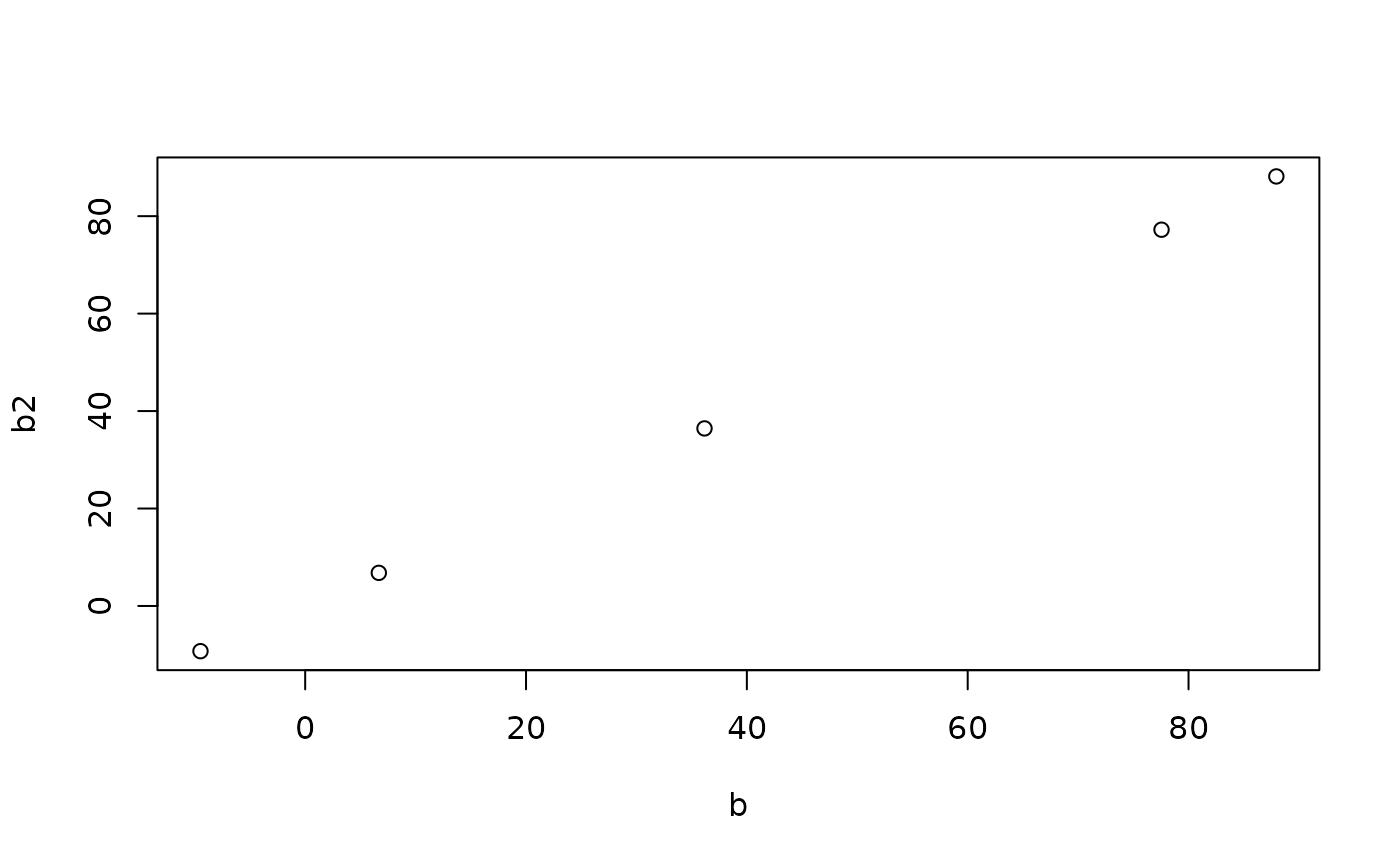This function returns the bearing (in degrees relative to north) of lines.

## Usage

``line_bearing(l, bidirectional = FALSE)``

## Arguments

l

A spatial lines object

bidirectional

Should the result be returned in a bidirectional format? Default is FALSE. If TRUE, the same line in the oposite direction would have the same bearing

## Details

Returns a boolean vector. TRUE means that the associated line is in fact a point (has no distance). This can be useful for removing data that will not be plotted.

Other lines: `angle_diff()`, `geo_toptail()`, `is_linepoint()`, `line2df()`, `line2points()`, `line_breakup()`, `line_midpoint()`, `line_segment1()`, `line_segment()`, `line_via()`, `mats2line()`, `n_segments()`, `n_vertices()`, `onewaygeo()`, `points2line()`, `toptail_buff()`

## Examples

``````l <- flowlines_sf[1:5, ]
bearings_sf_1_9 <- line_bearing(l)
bearings_sf_1_9 # lines of 0 length have NaN bearing
#>   -92.04942 -102.44566 -143.83660 -173.33154  170.51696
b <- line_bearing(l, bidirectional = TRUE)
r <- routes_fast_sf[1:5, ]
b2 <- line_bearing(r, bidirectional = TRUE)
plot(b, b2)``````